# Test: Graph Theory

## 10 Questions MCQ Test RRB JE for Electrical Engineering | Test: Graph Theory

Description
Attempt Test: Graph Theory | 10 questions in 30 minutes | Mock test for Railways preparation | Free important questions MCQ to study RRB JE for Electrical Engineering for Railways Exam | Download free PDF with solutions
QUESTION: 1

### Consider the following circuits :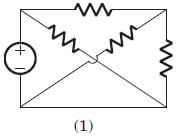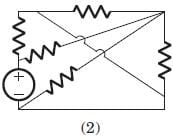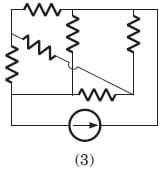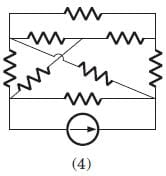The planner circuits are

Solution:

The circuit 1 and 2 are redrawn as below. 3 and 4 can not be redrawn on a plane without crossing other branch.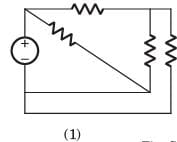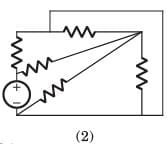QUESTION: 2

### Consider the following graphs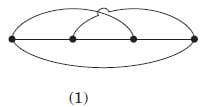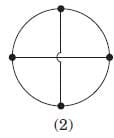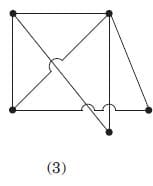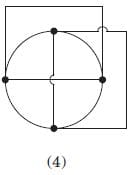Non-planner graphs are

Solution:

Other three circuits can be drawn on plane without crossing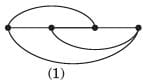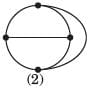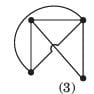QUESTION: 3

### A graph of an electrical network has 4 nodes and 7 branches. The number of links l, with respect to the chosen tree, would be

Solution:

l = b - (n - 1) = 4.

QUESTION: 4

For the graph shown in fig.correct set is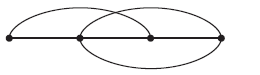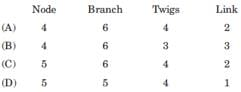Solution:

There are 4 node and 6 branches.

t = n - 1 = 3, l = b - n + 1 = 3

QUESTION: 5

A tree of the graph shown in fig. is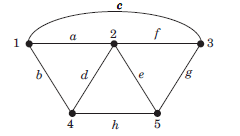Solution:

From fig. it can be seen that a f h g is a tree of given graph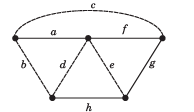QUESTION: 6

Branch current and loop current relation are expressed in matrix form as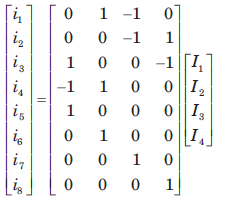where ij represent branch current and Ik loop current. The number of independent node equation are

Solution:

Number of branch = 8

Number of twigs = 8 - 4 = 4

Number of twigs = number of independent node equation.

QUESTION: 7

If the number of branch in a network is b, thenumber of nodes is n and the number of dependent loop is l, then the number of independent node equations will be

Solution:

The number of independent node equation are n - 1.

QUESTION: 8

Branch current and loop current relation are expressed in matrix form as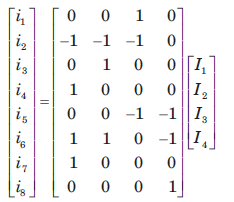.

where ij represent branch current and Ik loop current.

The rank of incidence matrix is

Solution:

Number of branch b = 8

Number of link l = 4

Number of twigs t = b - l = 4

rank of matrix = n - 1 = t = 4

QUESTION: 9

A network has 8 nodes and 5 independent loops. The number of branches in the network is

Solution:

l = b - (n - 1)

= 5 = b - 7

b = 12

QUESTION: 10

A branch has 6 node and 9 branch. The independent loops are

Solution:Use Code STAYHOME200 and get INR 200 additional OFF Use Coupon Code